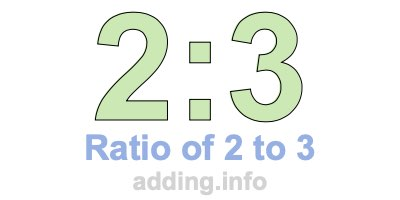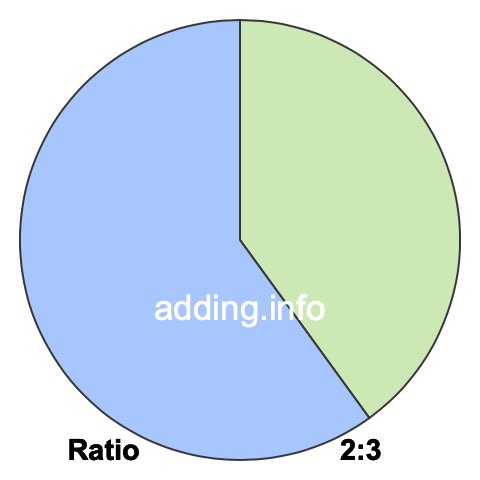Ratio of 2 to 3A ratio of 2 to 3 can be written as 2 to 3, 2:3, or 2/3. Furthermore, 2 and 3 can be the quantity or measurement of anything, such as students, fruit, weights, heights, speed and so on.

A ratio of 2 to 3 simply means that for every 2 of something, there are 3 of something else, with a total of 5.

In the box below, you can enter a number in either box and we will calculate the other number to keep the ratio of 2 to 3. In other words, we will calculate the corresponding equivalent ratio of 2:3 for you.

:

To calculate the left side of the ratio, our calculator multiplies the right side by 2 and then divides the product by 3. To calculate the right side of the ratio, our calculator multiplies the left side by 3 and then divides the product by 2. Answers are rounded to two decimals if necessary.

Below is a list of equivalent ratios of 2:3 that we created using our 2:3 ratio calculator above.

2:3

4:6

6:9

8:12

10:15

12:18

14:21

16:24

18:27

20:30

22:33

24:36

We have also created a pie chart for you to put 2:3 ratio in perspective. To do so, we divided a pie into 5 pieces and then colored 2 pieces green and 3 pieces blue. The pie will look exactly the same using any equivalent ratio of 2:3.The green part of the pie covers 40% and the blue part covers 60%. To calculate the percentages, we divided each side of the ratio by its total and then multiplied by 100, like this:

(2 / 5)×100 = 40
(3 / 5)×100 = 60

In fractional terms, 2/3 of the pie above is colored in green. This means that the ratio of 2 to 3 or 2:3 can also be expressed as a fraction, where the 2 to the left of the colon is the numerator and the 3 to the right of the colon is the denominator:

2:3 = 2/3

Ratio is often used when defining rectangles in width:height format. Width and height can be feet, inches, centimeters, or any other kind of length. With a ratio of 2 to 3, the width is 2 and the height is 3. Below is a picture of what a rectangle with a ratio of 2:3 looks like.Since the simplest form of the fraction 2/3 is 2/3, the simplest form of the ratio 2:3 is also 2:3.

Ratio Calculator
Need information about another ratio? Here you can submit another ratio for us to explain.

:

Ratio of 2 to 4
Go to the next ratio on our list that we have detailed information about.